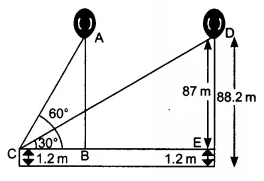Q

# A 1.2 m tall girl spots a balloon moving with the wind in a horizontal line at a height of 88.2 m from the ground. The angle of elevation of the balloon from the eyes of the girl at any instant is 60°. After some time, the angle of elevation reduces to 30

14. A 1.2 m tall girl spots a balloon moving with the wind in a horizontal line at a height of 88.2 m from the ground. The angle of elevation of the balloon from the eyes of the girl at any instant is 60°. After some time, the angle of elevation reduces to 30° (see Fig. 9.13). Find the distance travelled by the balloon during the interval.Views

Given that,
Height of the girl is 1.2 m. The height of the balloon from the ground is 88.2 m and the angle of elevation of the balloon from the eye of the girl at any instant is () and after some time .Let the  distance travelled by the balloon from position A to position D during the interval.
AB = ED = 88.2 - 1.2 =87 m

Now, In triangle ,

In triangle ,

Thus, distance travelled by the balloon from position A to D

m

Exams
Articles
Questions